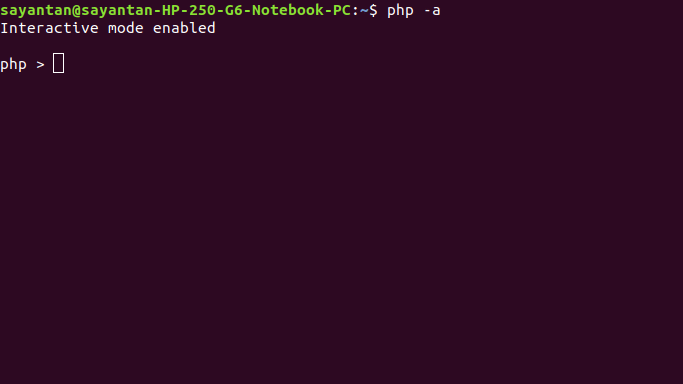# How to read user or console input in PHP ?

• Difficulty Level : Basic
• Last Updated : 21 May, 2022

In PHP, the console is a command-line interface, which is also called interactive shell. We can access it by typing the following command in a terminal:

`php -a`

If we type any PHP code in the shell and hit enter, it is executed directly and displays the output or shows the error messages in case of any error. A sample run of a PHP code, that reads input from PHP console looks like this:In this article, We will discuss two methods for reading console or user input in PHP:
Method 1: Using readline() function is a built-in function in PHP. This function is used to read console input.
The following things can be achieved by readline() function

• Accept a single input by prompting the user:

## PHP

 ``

Output:

```Enter a string: GeeksforGeeks
GeeksforGeeks```
• By default, the data type of the variable accepted through readline() function is string. So for any other data type, we have to typecast it explicitly as described below.

## PHP

 ``

Output:

```Enter an integer: 10
Enter a floating point number: 9.78
Entered integer is 10 and entered float is 9.78```
• We can achieve the same things without prompting the user also:
`\$a = readline();`
• In this case, as soon as the user hits enter, the entered value is stored in the variable a.
• Accept multiple space separated inputs: For this, we use another function explode() together with readline(). The first argument of explode() is the delimiter we want to use. In the below example, the delimiter is space. The second argument is the readline() function. Here also the data type of \$var1 and \$var2 will be string. So we have to separately typecast them for other data types. In the above example, the typecasting is shown for integers.

## PHP

 ``

Output:
The sum of 10 and 20 is 30

• We can also read an array through explode():

## PHP

 ` 1``    `` => 2``    `` => 3``    `` => 4``    `` => 5``    `` => 6``)*/`` ` `?>`

Output:

```Array
(
 => 1
 => 2
 => 3
 => 4
 => 5
 => 6
)```

Method 2: Using fscanf() function works same as the fscanf() function in C. We can read 2 integers from Keyboard(STDIN) as below:

• This is different from the previous method

## PHP

 ``

Output:

`The sum of 1 and 5 is 6`

Comparison between two methods:

• No need to use explicit typecasting for fscanf() function, because it is done by the format specifiers , e.g. %d, %f, %c etc. You can read more about format specifiers.
• fscanf() function is much faster than the readline() function.

PHP is a server-side scripting language designed specifically for web development. You can learn PHP from the ground up by following this PHP Tutorial and PHP Examples.

My Personal Notes arrow_drop_up